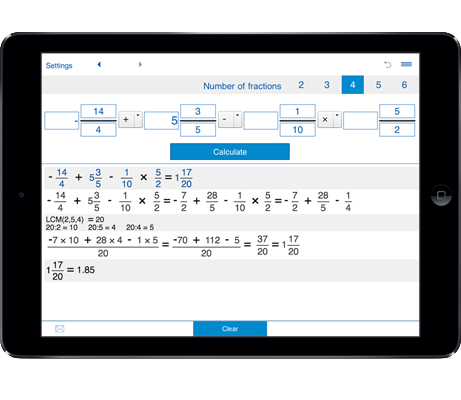# Dividing fractions

Fraction calculator with steps helps you calculate fractions, mixed numbers and whole numbers.
With fractions calculator you can divide any combination of fractions, mixed numbers, and whole numbers.

## Dividing fractions calculator

It is a handy fraction calculator to divide fractions, mixed fractions and whole numbers.

### Divide fractions, divide fractions with mixed numbers, divide fractions by whole numbers

You can use this fraction division calculator for dividing two, three, four, five, and six fractions, whole numbers and mixed numbers.

### How to use the dividing fractions calculator

Select the number of fractions for fraction division. You can select 2 fractions, 3 fractions, 4 fractions, 5 fractions, or 6 fractions.

Then enter the fractions in the provided fields and click "Calculate" to find the result.